## Use The Onion Method To Approach Complex Formulas

Complex Formulas? The Onion Method? Huh?

I’m talking about the idea that complex formulas in Google Sheets are a lot like onions.

They have layers.

And they sometimes make you cry. 🤣

## The Onion Method For Complex Formulas

If you’re building complex formulas, then I advocate following a one-action-per-step approach.

What I mean by this is that you build your formulas in a series of steps, and only make one change with each step.

The Onion Method is a framework by which to approach hard formulas, and consists of these three elements:

1. Put each new step of the formula in a new cell
2. Label each step with a simple “Step 1”, “Step 2”, etc. in adjacent cells
3. Change the background color of each formula cell, so they can be easily found

This lets you see the formula progress in an incremental way and is really helpful when you’re building or tyring to understand complex formulas.

Sometimes a step might result in an error (typically a #N/A or #REF!), but that’s ok, provided it gets fixed in a subsequent step, as shown in this SUMPRODUCT example: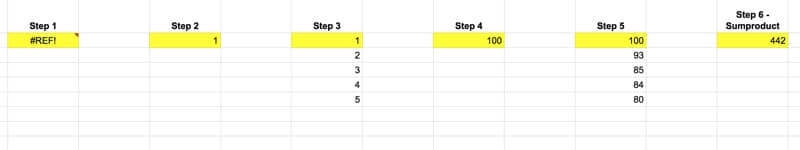Each of these intermediary formulas in the above image moves us forward incrementally, until the final answer is obtained in step 6.

Similarly, if you’re trying to understand complex formulas, peel the layers back until you reach the core (which is hopefully a function you understand!). Then, build it back up in steps to get back to the full formula.

## Example 1: Building Complex Formulas With The Onion Method

Let’s look at importing data from the table on this Wikipedia page of largest cities by population.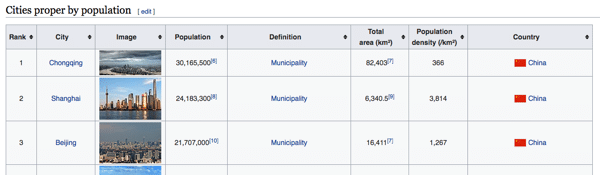### Step 0

Open a new Google Sheet (bonus points for using the browser shortcut and just typing `Sheets.new` into your browser window).

### Step 1

Step 1 is a standard IMPORTHTML function to retrieve the city table from Wikipedia. In cell A2:

`=IMPORTHTML("https://en.wikipedia.org/wiki/List_of_cities_proper_by_population","table",2)`

The data has some issues, but it’s a start.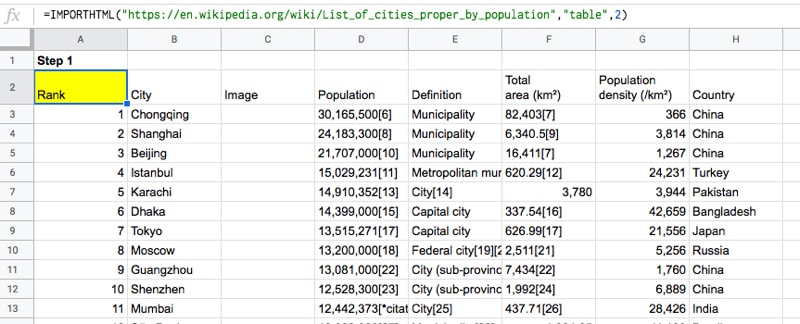### Step 2

In Step 2, we use the INDEX function to grab just the population column.

Per The Onion Method, we insert this next step in a new cell, cell J2, to the right of the existing data, in this example:

`=INDEX(IMPORTHTML("https://en.wikipedia.org/wiki/List_of_cities_proper_by_population","table",2),,4)`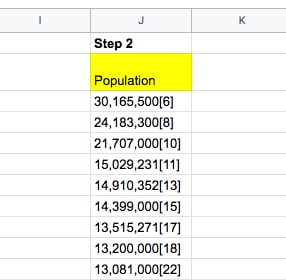I’ve highlighted the new action step in red — adding the INDEX function wrapper.

Looking closely at that INDEX function wrapper, you’ll see that we’ve left the row argument blank, namely:

`INDEX(data, ,4)`

which returns the entire column.

We’ll deal with the population column on its own and come back to our main formula later.

### Step 3

Hmm, that population column is messed up! Regex to the rescue!

Using the REGEXEXTRACT function, extract just the numbers and “,” from the data, before the citations in brackets:

`=REGEXEXTRACT(INDEX(IMPORTHTML("https://en.wikipedia.org/wiki/List_of_cities_proper_by_population","table",2),,4),"[0-9,]+")`

Hmm, that gives us a #N/A error… 🤔

### Step 4

Turn this into an Array Formula and get the column of population numbers!

`=ArrayFormula(REGEXEXTRACT(INDEX(IMPORTHTML("https://en.wikipedia.org/wiki/List_of_cities_proper_by_population","table",2),,4),"[0-9,]+"))`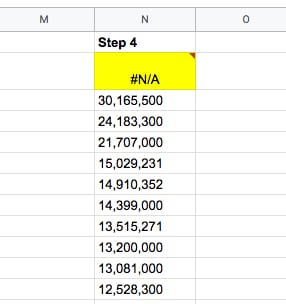We still have two problems to solve though: i) we need to convert the strings into actual numbers and ii) we need to fix the #N/A column heading…

### Step 5

We can multiply by 1 (see the “*1” at the end of our formula), which coerces those text strings into actual numbers.

`=ArrayFormula(REGEXEXTRACT(INDEX(IMPORTHTML("https://en.wikipedia.org/wiki/List_of_cities_proper_by_population","table",2),,4),"[0-9,]+") * 1)`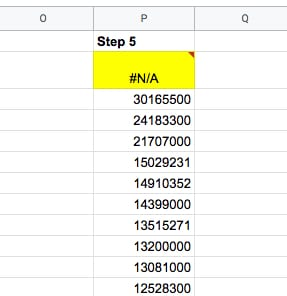### Step 6

Use the IFERROR function to fix that pesky #N/A error at the top of our column heading, and replace the #N/A with the word “Population”:

`=ArrayFormula(IFERROR(REGEXEXTRACT(INDEX(IMPORTHTML("https://en.wikipedia.org/wiki/List_of_cities_proper_by_population","table",2),,4),"[0-9,]+")*1,"Population"))`

Nice, now we have our population column as numbers:### Step 7

Pick the other columns we want, by wrapping the IMPORTHTML function with a QUERY function.

Note that we have to use the Col1 notation rather than the column letter in our Select statement, since we’re nesting another function as our data source in the QUERY function.

We also use the QUERY function rather than the INDEX function because we want to return multiple columns this time, which the INDEX function can’t do.

`=QUERY(IMPORTHTML("https://en.wikipedia.org/wiki/List_of_cities_proper_by_population","table",2),"SELECT Col2, Col8",1)`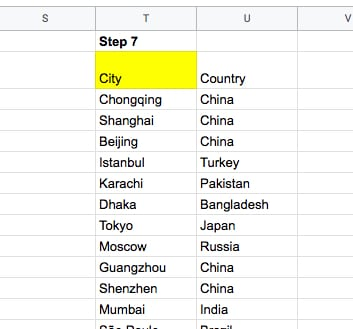### Step 8

All that’s left is to join these two ranges using the curly bracket notation, like this:

```={ QUERY(IMPORTHTML("https://en.wikipedia.org/wiki/List_of_cities_proper_by_population","table",2),"SELECT Col2,Col8",1) , ArrayFormula(IFERROR(REGEXEXTRACT(INDEX(IMPORTHTML("https://en.wikipedia.org/wiki/List_of_cities_proper_by_population","table",2),,4),"[0-9,]+") * 1,"Population")) ```

`}`

(Shown with line breaks to illustrate the two ranges.)

Ok, we’re done 🤪

The output is:## Example 2: Deconstructing Complex Formulas With The Onion Method

If you’re trying to understand complex formulas in Google Sheets that someone else has shared with you, you can still approach it with this Onion Method.

Simply peel back the layers until you reach the innermost function. Copy that into a new cell and start from the inside and work out, building up to the full formula again.

Let’s see an example.

Suppose we’re given this worksheet with US State names: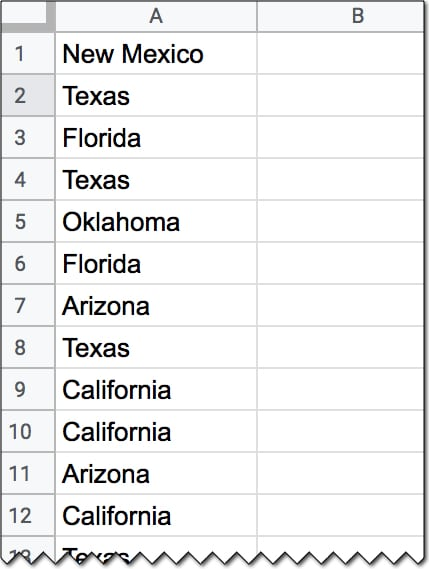And we’re also given this formula:

`=ArrayFormula(INDEX(A1:A20,MODE(MATCH(A1:A20,A1:A20,0))))`

which gives an output of Texas.

But how does this formula work?

Applying The Onion Method, we peel back the layers to the core function, and then build it up in steps again.

### Step 1

In a new cell, add the innermost MATCH function:

`=MATCH(A1:A20,A1:A20,0)`

### Step 2

`=ArrayFormula(MATCH(A1:A20,A1:A20,0))`

which outputs an array of the position of the first occurrence of the words in column A. We see a 2 next to every occurrence of Texas for example, because the first time it occurred was in position 2.

### Step 3

Now, we wrap it with the MODE function to find the most frequently occurring position:

`=ArrayFormula(MODE(MATCH(A1:A20,A1:A20,0)))`

By definition, the MODE function takes a range of numbers for an input and finds the most commonly occurring value.

However, what happens if we have a range of text values and want to find the most frequent?

In this case, the MATCH has been used to create a range of numbers for the MODE function.

By now, we’ve probably deduced that this formula finds the most frequent word in a list.

### Step 4

Finally, we can retrieve the actual text value, i.e. the most frequent State name, by adding the INDEX function to get the full original formula, like this:

`=ArrayFormula(INDEX(A1:A20,MODE(MATCH(A1:A20,A1:A20,0))))`

This will give the output Texas in this specific example.

Nice!

## Complex Formulas Onion Method Template For Your Use

This template contains both examples from this tutorial.

To make your own editable copy, please go to File > Make a copy… under the File menu.

## Complex Formulas Onion Method Conclusion

The Onion Method is a framework that allows you to approach complex formulas in a systematic way.

Even if you’re presented with an “impossible” challenge to answer or an “impossible” formula to decipher, just follow this framework. If required, peel back the layers and then work from the inside out in an incremental fashion.

You’ll be amazed at how quickly your understanding of challenging formulas broadens and deepens. You’ll encounter and understand brand new functions that you’ve never heard of before. Plus, you’ll find out all sorts of secret tricks with existing formulas.

Who knows, you might even cry tears of joy instead of despair…

I’ll leave you with this quote from businesswoman Belinda Johnson:

I like cutting through complexity and trying to get to the kernel of an idea.

## Formula Challenge #2: Matching Terms

(This Formula Challenge originally appeared as part of Google Sheets Tip #52, my weekly newsletter, on 27 May 2019.

Sign up here so you don’t miss out on future Formula Challenges!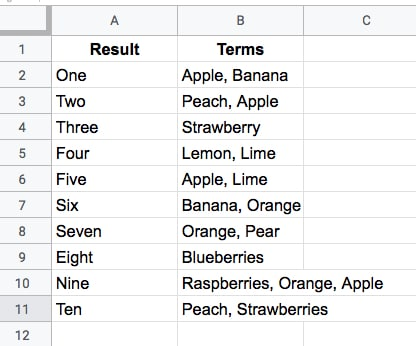Your challenge is to create a single-cell formula that takes a string of search Terms and returns all the Results that have at least one matching term in the Terms column.

For example, this search (in cell E2 say)

Raspberries, Orange, Apple

would return the results (in cell F2 say):

One
Two
Five
Six
Seven
Nine

like this (where the yellow is your formula):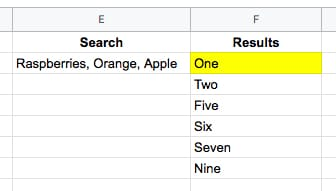## The Solution

### Solution One: Using the FILTER function

`=FILTER(A2:A11,REGEXMATCH(B2:B11,JOIN("|",SPLIT(E2,", "))))`

or even:

`=FILTER(A2:A11,REGEXMATCH(B2:B11,SUBSTITUTE(E2,", ","|")))`

These elegant solutions were also the shortest solutions submitted.

There were a lot of similar entries that had an ArrayFormula function inside the Filter, but this is not required since the Filter function will output an array automatically.

How does this formula work?

Let’s begin in the middle and rebuild the formula in steps:

`=SPLIT(E2,", ")`

splits out the three fruits in cell E2 into separate cells:

`Raspberries    Orange    Apple`

Next, join them back together with the pipe “|” delimiter with

`=JOIN("|",SPLIT(E2,", "))`

so the output is now:

`Raspberries|Orange|Apple`

Then bring the power of regular expression to the table, to match the data in column B. The pipe character means “OR” in regular expressions, so this formula will match Raspberries OR Orange OR Apple in column B:

`=REGEXMATCH(B2:B11,JOIN("|",SPLIT(E2,", ")))`

On its own, this formula will return a #VALUE! error message. (Wrap this with the ArrayFormula function if you want to see what the array of TRUE and FALSE values looks like.)

However, when we put this inside of a FILTER function, the correct array value is passed in:

`=FILTER(A2:A11,REGEXMATCH(B2:B11,JOIN("|",SPLIT(E2,", "))))`

and returns the desired output. Kaboom! 💥

### Solution Two: Using the QUERY function

`=QUERY(A2:B11,"select A where B contains '"&JOIN("' or B contains '",SPLIT(E2,", "))&"'")`

As with solution one, there is no requirement to use an ArrayFormula anywhere. Impressive!

This formula takes a different approach to solution one and uses the QUERY function to filter the rows of data.

The heart of the formula is similar though, splitting out the input terms into an array, then recombining them to use as filter conditions.

`=JOIN("' or B contains '",SPLIT(E2,", ",0))`

which outputs a clause ready to insert into your query function, viz:

`Raspberries' or B contains 'Orange' or B contains 'Apple`

The QUERY function uses a pseudo-SQL language to parse your data. It returns rows from column A, whenever column B contains Raspberries OR Orange OR Apple.

Wonderful!

I hope you enjoyed this challenge and learnt something from it. I really enjoyed reading all the submissions and definitely learnt some new tricks myself.

### SPLIT function caveats

There are two dangers with the Split function which are important to keep in mind when using it (thanks to Christopher D. for pointing these out to me).

Caveat 1

The SPLIT function uses all of the characters you provide in the input.

So

`=SPLIT("First sentence, Second sentence", ", ")`

will split into FOUR parts, not two, because the comma and the space are used as delimiters. The output will therefore be:

`First    sentence    Second    sentence`

across four cells.

Caveat 2

Datatypes may change when they are split, viz:

`=SPLIT("Lisa, 01",",")`

gives an output of

`Lisa    1`

where the string has been converted into a number, namely 1.

See the other Formula Challenges here.

## Introduction

I firmly believe that one of the most effective and rewarding ways to learn a skill is through practical application.

Solving problems you don’t know the answer to is arguably the best way to do this.

And that’s the idea behind these Formula Challenges.

I’ll post a challenge in my Monday newsletter — a question to be solved with formulas in Google Sheets — and a week later share solutions, both my own and those submitted by readers.

I’ll archive the challenges and solutions on my website here.

(This first Formula Challenge originally appeared in my Google Sheets Tips newsletter, on 25 February 2019.

Sign up here so you don’t miss out on future Formula Challenges!)

## The Challenge

`=IMAGE("https://www.google.com/favicon.ico")`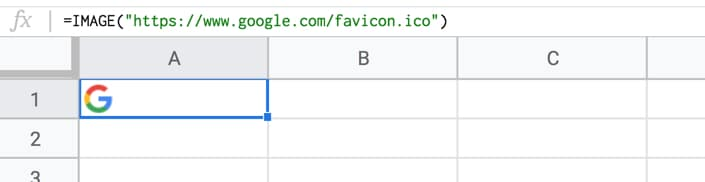Your challenge is to modify the formula in cell A1 only, to repeat the image across multiple columns (say 5 as in this example), so it looks like this: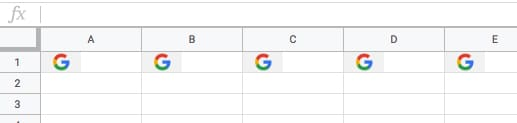Rules

You’re only allowed to use a single formula in cell A1.

The problem is that the IMAGE function can’t be nested inside a REPT function, so you have to get a bit more creative.

## The Solution

### Solution One: using ROW or COLUMN counts

`=ArrayFormula(IF(COLUMN(A:E),IMAGE("https://www.google.com/favicon.ico")))`

#### How does this formula work?

The combination of ArrayFormula with COLUMN(A:E) will output an array of numbers 1 to 5: `{1,2,3,4,5}`

The IF statement treats the numbers as TRUE values, so prints out the image 5 times. For brevity, we can omit the FALSE value of the IF statement, since we don’t call it.

### Solution Two: using REPT inside the IMAGE formula!

`=ArrayFormula(IMAGE(SPLIT(REPT("https://www.google.com/favicon.ico"&"|",5),"|")))`

#### How does this formula work?

As mentioned, the REPT function doesn’t work when wrapped around the IMAGE function. However, flip them around, with the REPT inside the IMAGE function, and it does work!

In other words the IMAGE function accepts arrays of URLs as an input.

Start with this formula in cell A1, which creates a single string of joined URLs, with a pipe ( | ) delimiter between them:

`=ArrayFormula(REPT("https://www.google.com/favicon.ico"&"|",5))`

Now, split these into an array of 5 separate URLs:

`=ArrayFormula(SPLIT(REPT("https://www.google.com/favicon.ico"&"|",5),"|"))`

Finally, wrap this with the IMAGE function to get the five images in a row:

`=ArrayFormula(IMAGE(SPLIT(REPT("https://www.google.com/favicon.ico"&"|",5),"|")))`

What I like about this solution is that you could put the number 5 into a different cell and reference it, so that you can easily change how many times the image is repeated.

You could even embed another formula to calculate how many times to repeat the image 😉

See the other Formula Challenges here.